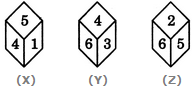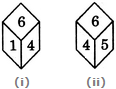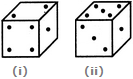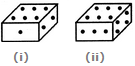# Non Verbal Reasoning - Cubes and Dice

### Exercise :: Cubes and Dice - Section 2

16.

Three different positions X, Y and Z of a dice are shown in the figures given below. Which number lies at the bottom face in position X?A. 2 B. 3 C. 6 D. Cannot be determined

Answer: Option B

Explanation:

From positions X and Y we conclude that 1, 5, 6 and 3 lie adjacent to 4. Therefore, 2 must lie opposite 4. From positions Y and Z we conclude that 4, 3, 2 and 5 lie adjacent to 6. Therefore, 1 must lie opposite 6. Thus, 2 lies opposite 4, 1 lies opposite 6 and consequently 5 lies opposite 3.

As analysed above, the number on the face opposite 5 is 3. In position X, since 5 lies on the top, therefore 3 must lie at the bottom face.

17.

A dice is numbered from 1 to 6 in different ways.

If 2 is opposite to 3 and adjacent to 4 and 6, then which of the following statements is necessarily true?

 A. 1 is opposite to 5 B. 4 is opposite to 6 C. 4 is adjacent to 2 and 6 D. 1 is adjacent to 2 and 3

Answer: Option D

Explanation:

If 2 is opposite to 3, then 1 cannot lie opposite to either of the two numbers - 2 or 3. Hence, 1 is necessarily adjacent to both 2 and 3.

18.

Two positions of a dice are shown below. When number 1 is on the top, what number will be at the bottom?A. 2 B. 3 C. 5 D. Cannot be determined

Answer: Option C

Explanation:

Number 6 is common to both the positions of the dice. We assume the dice in fig. (ii) to be rotated so that 6 remains on the top face (i.e. face V as per activity 1) and the number 4 in fig. (ii) moves to the FR-RH face (i.e. face I) as in fig. (i), then 5 will move to the RR-RH face (i.e. face II). Clearly, 5 (which lies on face II) and 1 (which lies on face IV) will be opposite to each other. So, when 1 is on the top, then 5 will be at the bottom.

19.

Two positions of a cube are shown below. When the number 4 will be at the bottom, then which number will be at the top?A. 3 B. 5 C. 6 D. Cannot be determined

Answer: Option A

Explanation:

Number 1 is common to both the positions of the dice. We assume the dice in fig. (ii) to be rotated so that 1 remains on the RHS face (i.e. face II as per activity 1) and the numbers 5 and 3 move to the faces hidden behind 2 and 4 respectively (in fig. (i). Then, clearly 3 lies opposite 4. Hence, when 4 is at the bottom then 3 mist lie on the top.

20.

Two positions of a block are shown below: When six is at the bottom, what number will be at the top?A. 1 B. 2 C. 4 D. 5

Answer: Option D

Explanation:

From figures (i) and (ii) we conclude that the number 1, 2, 3 and 4 appear adjacent to 6. Thus, the number 5 will appear opposite 6. Therefore, when six is at the bottom, then 5 will be at the top.

#### Current Affairs 2021

Interview Questions and Answers